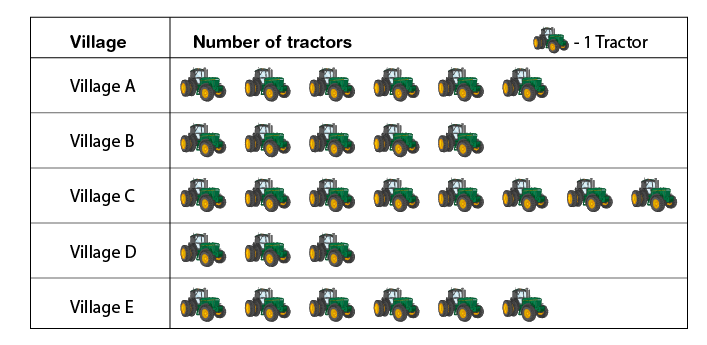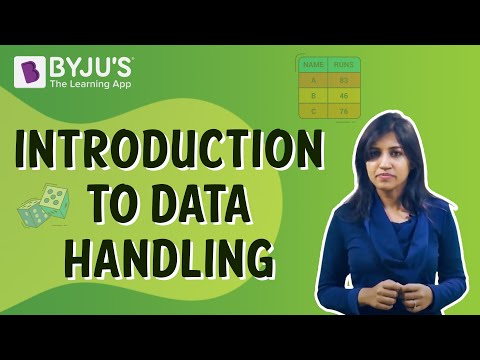# Data Handling Worksheets

Data handling worksheets include practice questions based on the data handling concepts for students of Class 6, Class 7 and Class 8. These worksheets are very helpful to the revision of the data handling chapter.

We solve so many problems mentally in daily life whether it is about billed payments, date calculation or simple data handling like the number of students in a class per number of students. We never realize their importance since data handling has become a part of our daily life calculations.

When it comes to data handling worksheets, we solve numerous types of problems that include frequency distribution, fractions worksheets and grouping data from NCERT textbooks along with prescribed reference books. Data handling forms a base of every problem based on Statistics whether it is Population concept or average marks obtained by the total number of students.

## Data Handling Worksheet for Class 6

Q.1: What is the quickest way of recording data?

Q.2: ______ is the representation of data using pictures.

Q.3: With the help of a bar graph and using a suitable scale, show the data of the population of a city.

 Men 1000 Women 1200 Children 1500 Senior citizens 700

Q.4: In a Mathematics test, marks obtained by 40 students are given below in the table. Arrange these marks in a table using tally marks.

 8 1 3 7 6 5 5 4 4 2 4 9 5 3 7 1 6 5 2 7 7 3 8 4 2 8 9 5 8 6 7 4 5 6 9 6 4 4 6 6
• Find how many students obtained marks equal to or more than 5.
• How many students obtained marks below 3?

Q.5: A pictograph shows the number of tractors in five villages. Observe the pictograph and answer the following questions.• Which village has the minimum number of tractors?
• Which village has the maximum number of tractors?

## Data Handling Worksheet for Class 7

Q.1: Find the range of the following data: 30, 15, 16, 18, 10, 24, 26, 20.

Q.2: What is the mode of following data?

24, 26, 23, 26, 22, 25, 26, 28

Q.3: What is the median of the following data?

20, 14, 6, 25, 18, 13, 19, 10, 9, 12

Q.4: A fair die is rolled, find the probability of getting an odd number.

Q.5: Find the mean and median of the first ten prime numbers.

## Data Handling Worksheet for Class 8

Q.1 What are the possible outcomes of the following?

• Tossing a coin
• Tossing three coins
• Throwing a two dice

Q.2: Construct a frequency distribution table for the data on weights (in kg.) of 20 people in a group, using intervals 30-40, 35-40 and so on.

40, 38, 33, 48, 60, 53, 31, 46, 34, 36, 49, 41, 55, 49, 55, 42, 44, 47, 47, 38, 39.

Q.3: A pack of 52 cards is shuffled and a card is drawn without seeing. What is the probability that the card drawn is:

• An Ace of club
• A Heart
• A Face card
• Black
• A jack
• Not a face card

Q.4: The following data shows the expenditure of a person on different things during a month. Draw a pie chart to represent the data.

 Items Expenditure (in Rs.) Rent of house 8000 Electricity bill 1600 Food 3000 Phone bill 900 Others 4500

Q.5: What is the probability of a number selected from the numbers 1, 2, 3, ….., 20 such that it is a composite number?

## Video Lesson on Data Handling